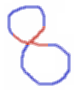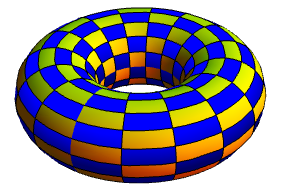# Topological tacks

If you’ve bought a box of drawing pins from a stationery store, you’ll observe that the pins do not pack tightly at all, and there is lots of empty space in there. Indeed, it’s impossible to pack them particularly efficiently, due to an interesting theorem in topology. We’ll begin by considering the old puzzle that asks whether we can fit uncountably many topological lemniscates in the plane. Here are some examples of topological lemniscates in the plane:We can certainly get a countably infinite set of disjoint topological lemniscates, by nesting them inside each other, analogous to Russian dolls. After some initial thought, you can deduce that there are no uncountable sets of disjoint topological lemniscates; each ‘lobe’ must contain a rational point, so we can define a function from the set of lemniscates to the set Q² × Q² of ordered pairs of rational points by choosing an arbitrary point in each lobe. This function must be injective, as otherwise the lemniscates must intersect. There are only countably many ordered pairs of rational points, completing the proof.

A variant of this problem is whether there exists a set of uncountably many disjoint topological Y-shapes in the plane. This is strictly harder since a topological lemniscate is necessarily a superset of a topological Y-shape:Again, the answer is still no. James Aaronson showed me an elegant proof, involving the non-planarity of the complete bipartite graph K3,3. We map each Y-shape to a triple of rational circles, each one of which contains precisely one of the three endpoints and does not contain the centre. This isn’t quite an injection, as two disjoint Y-shapes can map to the same triple of rational circles:However, three disjoint Y-shapes cannot map to the same triple of rational circles, as it would imply that the complete bipartite graph K3,3 is planar. It’s trivial to prove that this is false, with a little help from the Jordan Curve Theorem.

What about the surface of a torus? K3,3 is a toroidal graph (indeed, it’s possible to draw K7 on the torus without edges crossing), so the argument breaks down. It can be repaired, however, by covering the torus with a finite collection of overlapping simply-connected regions, each of which can only contain countably many topological Y-shapes.In the example above, each region is a 2-by-2 block containing two blue and two yellow squares. The centre of every Y-shape must lie in the interior of at least one region (even if it lies on the boundary between two squares). We can apply this argument to any topological surface of finite genus to show that only countably many topological Y-shapes can inhabit it.

There is actually a generalisation to R^n. A tack is the union of a line segment and an open (n−1)-ball, which intersect at a single point (an endpoint of the line segment, which lies in the interior of the ball). It has been proved that there does not exist an uncountable set of disjoint topological tacks in R^n for any n. This implies that they can’t be packed tightly, which is why there is lots of empty space left over in the box of drawing pins.

This entry was posted in Uncategorized. Bookmark the permalink.

### 0 Responses to Topological tacks

1.Johnicholas says:

Forgive my obtuseness – are you making a funny-because-it’s-obviously-false implication “there does not exist an uncountable set of disjoint X” to “X do not pack particularly densely in practice”? Or is that a real, pragmatic principle?

For example, identical cubical dice might not pack densely in my dice bag, but under some circumstances (shaking, magnets) they probably do – there are cubical diamond and silicon crystals, after all. But the real world “dense” structure isn’t uncountably dense, it’s clearly countable via triples of natural numbers.

•apgoucher says:

Drawing pins have negligible thickness compared with their diameter. Let the thickness tend to zero. If we could pack them efficiently (i.e. exceed a given density), then we would need to fill a nonzero volume with a union of drawing pins, each of which has zero volume. The measure (in this case, volume) of a set is countably additive, so we need an uncountable number of drawing pins to achieve this.

This argument doesn’t apply to the situation with cubic dice, as the dice have nonzero volume.

2.Kasuha says:

The rules don’t seem to prohibit this:

http://i47.tinypic.com/24yakoz.png

But I guess the trick is in setting up small enough circles – for each end, find smallest distance to any other lemniscate, and set up the circle radius at 1/3 of that value.
Or can we afford doing that?

•apgoucher says:

Oh, sorry, I omitted the crucial detail that only one of the three arms is allowed to enter each circle.

3.Kasuha says: